# Texas Go Math Grade 4 Lesson 16.4 Answer Key Multiply and Divide Money

Refer to our Texas Go Math Grade 4 Answer Key Pdf to score good marks in the exams. Test yourself by practicing the problems from Texas Go Math Grade 4 Lesson 16.4 Answer Key Multiply and Divide Money.

## Texas Go Math Grade 4 Lesson 16.4 Answer Key Multiply and Divide Money

Essential Question

How can you use multiplication and division in problems involving money?
Multiplying and dividing money is just like multiplying and dividing whole numbers.

Unlock the Problem

Example 1 Mrs. Cleary wants to buy books for her bookstore. The new novel she wants comes in a case containing 48 books. Each book costs her $15, How much would 1 case of the books cost? Math Idea: Multiplying and dividing money is just like multiplying and dividing whole numbers. Use place value and regrouping. Multiply. 48 ×$15

STEP 1
Think of 48 as 4 tens and 8 ones. Multiply $15 by 8 ones, or 8.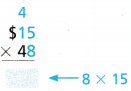Answer: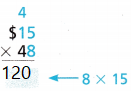STEP 2 Multiply 15 by 4 tens, or 40.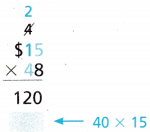Answer: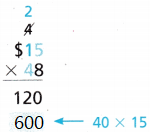STEP 3 Add the partial products Place a dollar sign in the final product.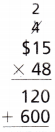Answer: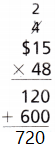So, Mrs. Cleary will pay _____________ for 1 case of the books. Explanation: So, Mrs. Cleary will pay 720 for 1 case of the books. Example 2 Mrs. Cleary paid$584 for 8 cases of a popular magazine. How much did she pay for each case of magazines?

Divide. $584 ÷ 8 STEP 1 Divide the tens.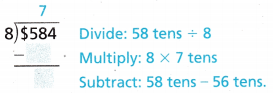Answer: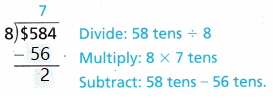STEP 2 Divide the ones. Regroup 2 tens as 20 ones.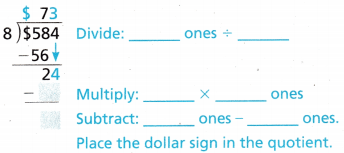Answer: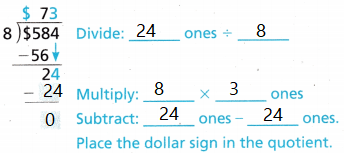So, Mrs. Cleary paid ______________ for each case of magazines. Explanation: So, Mrs. Cleary paid 73 for each case of magazines. Share and Show Question 1. Ray bought 18 baseball caps for his baseball team. Each cap costs$29. How much did Ray spend on caps?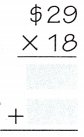So, Ray spent _____________ on caps.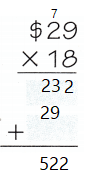Explanation:
So, Ray spent 522 on caps.

Question 2.
Samantha sold 9 teddy bears that she made. She was paid a total of $126 for all the bears. If the bears were all the same price, what was the price of one bear? Answer: 14 was the price of one bear Explanation: Samantha sold 9 teddy bears that she made. She was paid a total of$126 for all the bears.
126 ÷ 9 = 14

Question 3.
Harley is planning a special dinner for the debate club. There are 21 members in the club. If each member’s dinner costs $16, what will the total cost of the meals be? Answer: 336 the total cost of the meals be Explanation: There are 21 members in the club. If each member’s dinner costs$16,
21 x 16 = 336

Problem Solving

Practice: Copy and Solve

Question 4.
24 × $42 Answer:24 x 42 = 1008 Explanation: Found the product of algebraic multiplication. Question 5.$453 ÷ 3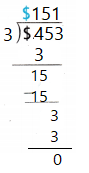453 ÷ 3 = 151
Explanation:
Found the product of algebraic Division.

Question 6.
68 × $92 Answer: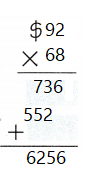68 ×$92 = 6256
Explanation:
Found the product of algebraic multiplication.

Question 7.
$875 ÷ 7 Answer:875 ÷ 7= 125 Explanation: Found the product of algebraic Division. Question 8.$6,295 ÷ 56,295 ÷ 5 = 1259
Explanation:
Found the product of algebraic Division.

Question 9.
7 × $1,372 Answer: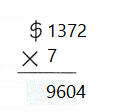7 ×$1,372 = 9604
Explanation:
Found the product of algebraic multiplication.

Question 10.
H.O.T. Palo bought a car for $1,284 using money from his savings account. He wants to repay his savings account over the next 6 months. If he saves an equal amount each month, how much will he need to save each month? Answer:$214 he need to save each month
Explanation:
Palo bought a car for $1,284 using money from his savings account. He wants to repay his savings account over the next 6 months. 1284 ÷ 6 = 214 Question 11. H.O.T. Multi-Step Lassa bought a car. She paid the dealer$2,220 and then finished paying for her car by making 9 equal payments of $352. How much was the total cost of her car? Answer:$5406 was the total cost of her car
Explanation:
9 x 352 = 3186
2220 + 3186 = 5406

Problem Solving

Question 12.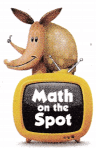H.O.T. Multi-Step Don buys a shirt for $24.85. His friend Daryl spends$3.15 more for his shirt. Sean paid the same price as Daryl for his shirt but bought 2. How much did Sean spend for his 2 shirts?
$28 Sean spend for his 2 shirts Explanation: Don buys a shirt for$24.85.
His friend Daryl spends $3.15 more for his shirt. Daryl and Sean are same 24.85 + 3.15 = 28 Question 13. H.O.T. Multi-Step Stacy buys 3 CDs in a set for$29.75. She saved $6.25 by buying the set instead of buying the CDs separately. If each CD costs the same amount, how much does each of the 3 CDs cost when purchased separately? Answer: 12 is each of the 3 CDs cost when purchased separately Explanation: 29.75 + 6.25 = 36 36 ÷ 3 = 12 Question 14. H.O.T. Multi-Step Julie saved$60. Jeff saved 29 times as much as Julie did. Jeff saved 3 times as much as Marco. How much did Marco save? Explain how you found your answer.
5220 that Marco save
Explanation:
60 x 29 = 1740
1740 x 3 = 5220

Question 15.
H.O.T. Multi-Step The grocery store has a sale on soup. One can costs $2.59 but you can buy 8 cans for a total of$16.00. How much do you save per can if you buy 8 at the sale price?
$0.59 save per can if you buy 8 at the sale price Explanation: 8 x 2.59 = 20.72 20.72 – 16.00= 4.72 4.72 ÷ 8 = 0.59 Question 16. Apply Billy plans to save$55 each month for 5 years. How much will he have at the end of 5 years?
$3300 he have at the end of 5 years Explanation: 1 year = 12 months 5 x 12 = 60 60 x 55 =$3300.

Question 17.
Jen downloads 7 apps for a total of $24. Two of them cost$2.00 each. If the other apps all cost the same amount, how much does each cost?
(A) $3.43 (B)$3.70
(C) $4 (d)$4.25
Answer: $4 Explanation: 24 – 4 = 20 20 divided by 5 = 4 each app costs. Question 18. Multi-Step Jeremiah spends$51.25 at the mall. He buys a game for $24.25 and 3 DVDs. If the DVDs are all the same price, how much does 1 DVD cost? (A)$15
(B) $8 (C)$25
(D) $9 Answer: D Explanation: 51.25 – 24.25 = 27 27 divided by 3 = 9 1 DVD cost =$9

Question 19.
Multi-Step Delilah buys a loaf of bread, 3 pounds of turkey, and 2 pounds of cheese. The turkey costs $3.00 per pound and the cheese costs$2.00 per pound. A loaf of bread costs $3.49. How much does Delilah spend in all? (A)$13.75
(B) $16.49 (C)$8.49
(D) $17.49 Answer: B Explanation: 3 x 3 = 9 2 x 2 = 4 3.49 + 9 + 4 = 16.49 Delilah spend in all TEXAS Test Prep Question 20. While working at the school store, John sold a letter jacket for$40.50 and some notebooks for $2.00 each. If he collected a total of$92.50, how many notebooks did he sell?
(A) 25
(B) 66
(C) 26
(D) 104
Explanation:
92 – 40 = $52 52 divided by 2 = 26 He sell 26 note books. ### Texas Go Math Grade 4 Lesson 16.4 Homework and Practice Answer Key Solve Question 1. 26 ×$44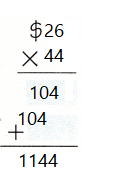26 × $44 = 1144 Explanation: Found the product of algebraic Multiplication. Question 2.$318 ÷ 6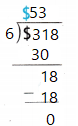318 ÷ 6 = 53
Explanation:
Found the product of algebraic Division.

Question 3.
35 × $73 Answer:35 ×$73 = 2555
Explanation:
Found the product of algebraic Multiplication.

Question 4.
$572 ÷ 4 Answer: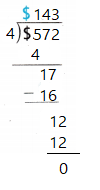$572 ÷ 4 = 143
Explanation:
Found the product of algebraic Division.

Question 5.
$7,640 ÷ 5 Answer:$7,640 ÷ 5 = 1528
Explanation:
Found the product of algebraic Division.

Question 6.
6 × $1,336 Answer:6 ×$1,336 = 8016
Explanation:
Found the product of algebraic Multiplication.

Problem Solving

Question 7.
Multi-Step Margie bought 3 identical sweaters for a total of $114. She saved$18 by buying the 3 sweaters on sale rather than at the regular price. What is the regular price of each sweater? Explain how you found the answer.
$44 is the regular price of each sweater Explanation: 114 + 18 =$132
$132 divided by 3 =$44

Question 8.
Multi-Step Keira rented a car for 3 days. She paid $118 rent each day. Insurance for the 3 days came to a total of$35. How much did Keira pay in all? Explain how you found your answer.
389 is the amount Keira pay in all.
Explanation:
3 x 118 = 354
354 + 35 = 389

Lesson Check

Question 9.
A box of 5 model race cars costs $115. How much does each model car cost? (A)$ 23
(B) $25 (C)$13
(D) $15 Answer: A Explanation: 115 divided by 5 =$23
$23 each model car cost Question 10. Jonathan bought 3 airplane tickets for$478 each. How much did he pay for the three tickets?
(A) $1,234 (B)$1,434
(C) $1,414 (D)$1,214
Explanation:
3 x 478 = 1,434
1,434 he payed for the three tickets

Question 11.
Multi-Step The Wallace family saves the same amount of money each month for vacation. From January 1 to September 30 they saved $1,314. How much does the Wallace family save each month for vacation? (A)$156
(B) $131 (C)$146
(D) $152 Answer: C Explanation: 1,314 divided by 9$146 the Wallace family save each month for vacation

Question 12.
Multi-Step A coach bought 3 dozen soccer balls. Each ball cost $28. How much did the coach pay for all of the soccer balls? (Remember, 1 dozen = 12.) (A)$84
(B) $840 (C)$336
(D) $1,008 Answer: D 3 x 12 = 36 36 x 28 =$1008 the coach pay for all of the soccer balls

Question 13.
Multi-Step Tony spent $49 at the fair. Molly spent$14 more than twice as much as Tony spent. How much did the two of them spend in all?
(A) $112 (B)$161
(C) $126 (D)$140
77 + 49 = $126 the two of them spend in all. Question 14. Multi-Step Paula bought 8 pairs of socks. She paid$104. Two pairs of socks cost $16 each. The other pairs each cost the same amount. How much did each of the other pairs of socks cost? (A)$12
(B) $17 (C)$13
(D) $18 Answer: A Explanation: 104 – 32 = 72 72 divided by 6 =$12 each of the other pairs of socks cost